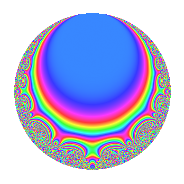# Properties

 Label 15.5.cLevel 15 Weight 5 Character orbit c Rep. character $$\chi_{15}(11,\cdot)$$ Character field $$\Q$$ Dimension 6 Newforms 1 Sturm bound 10 Trace bound 0

# Related objects

## Defining parameters

 Level: $$N$$ = $$15 = 3 \cdot 5$$ Weight: $$k$$ = $$5$$ Character orbit: $$[\chi]$$ = 15.c (of order $$2$$ and degree $$1$$) Character conductor: $$\operatorname{cond}(\chi)$$ = $$3$$ Character field: $$\Q$$ Newforms: $$1$$ Sturm bound: $$10$$ Trace bound: $$0$$

## Dimensions

The following table gives the dimensions of various subspaces of $$M_{5}(15, [\chi])$$.

Total New Old
Modular forms 10 6 4
Cusp forms 6 6 0
Eisenstein series 4 0 4

## Trace form

 $$6q$$ $$\mathstrut +\mathstrut 8q^{3}$$ $$\mathstrut -\mathstrut 50q^{4}$$ $$\mathstrut -\mathstrut 2q^{6}$$ $$\mathstrut +\mathstrut 76q^{7}$$ $$\mathstrut +\mathstrut 118q^{9}$$ $$\mathstrut +\mathstrut O(q^{10})$$ $$6q$$ $$\mathstrut +\mathstrut 8q^{3}$$ $$\mathstrut -\mathstrut 50q^{4}$$ $$\mathstrut -\mathstrut 2q^{6}$$ $$\mathstrut +\mathstrut 76q^{7}$$ $$\mathstrut +\mathstrut 118q^{9}$$ $$\mathstrut +\mathstrut 50q^{10}$$ $$\mathstrut -\mathstrut 452q^{12}$$ $$\mathstrut -\mathstrut 424q^{13}$$ $$\mathstrut +\mathstrut 50q^{15}$$ $$\mathstrut +\mathstrut 802q^{16}$$ $$\mathstrut +\mathstrut 1160q^{18}$$ $$\mathstrut -\mathstrut 244q^{19}$$ $$\mathstrut -\mathstrut 876q^{21}$$ $$\mathstrut +\mathstrut 340q^{22}$$ $$\mathstrut -\mathstrut 786q^{24}$$ $$\mathstrut -\mathstrut 750q^{25}$$ $$\mathstrut -\mathstrut 352q^{27}$$ $$\mathstrut -\mathstrut 3764q^{28}$$ $$\mathstrut +\mathstrut 2200q^{30}$$ $$\mathstrut +\mathstrut 3772q^{31}$$ $$\mathstrut +\mathstrut 4420q^{33}$$ $$\mathstrut +\mathstrut 3124q^{34}$$ $$\mathstrut -\mathstrut 7606q^{36}$$ $$\mathstrut +\mathstrut 1896q^{37}$$ $$\mathstrut -\mathstrut 1336q^{39}$$ $$\mathstrut -\mathstrut 4650q^{40}$$ $$\mathstrut -\mathstrut 1980q^{42}$$ $$\mathstrut -\mathstrut 7384q^{43}$$ $$\mathstrut +\mathstrut 1900q^{45}$$ $$\mathstrut +\mathstrut 8196q^{46}$$ $$\mathstrut +\mathstrut 14668q^{48}$$ $$\mathstrut -\mathstrut 1318q^{49}$$ $$\mathstrut -\mathstrut 8492q^{51}$$ $$\mathstrut +\mathstrut 8976q^{52}$$ $$\mathstrut -\mathstrut 278q^{54}$$ $$\mathstrut -\mathstrut 1300q^{55}$$ $$\mathstrut -\mathstrut 11584q^{57}$$ $$\mathstrut -\mathstrut 23740q^{58}$$ $$\mathstrut +\mathstrut 5050q^{60}$$ $$\mathstrut +\mathstrut 6452q^{61}$$ $$\mathstrut +\mathstrut 14796q^{63}$$ $$\mathstrut +\mathstrut 3174q^{64}$$ $$\mathstrut -\mathstrut 12760q^{66}$$ $$\mathstrut +\mathstrut 13816q^{67}$$ $$\mathstrut +\mathstrut 5472q^{69}$$ $$\mathstrut -\mathstrut 2100q^{70}$$ $$\mathstrut -\mathstrut 2040q^{72}$$ $$\mathstrut +\mathstrut 596q^{73}$$ $$\mathstrut -\mathstrut 1000q^{75}$$ $$\mathstrut +\mathstrut 21348q^{76}$$ $$\mathstrut -\mathstrut 1400q^{78}$$ $$\mathstrut -\mathstrut 16124q^{79}$$ $$\mathstrut +\mathstrut 5086q^{81}$$ $$\mathstrut -\mathstrut 31240q^{82}$$ $$\mathstrut -\mathstrut 14736q^{84}$$ $$\mathstrut -\mathstrut 3100q^{85}$$ $$\mathstrut -\mathstrut 4900q^{87}$$ $$\mathstrut +\mathstrut 15660q^{88}$$ $$\mathstrut +\mathstrut 7550q^{90}$$ $$\mathstrut -\mathstrut 11632q^{91}$$ $$\mathstrut -\mathstrut 8184q^{93}$$ $$\mathstrut +\mathstrut 34924q^{94}$$ $$\mathstrut +\mathstrut 14354q^{96}$$ $$\mathstrut +\mathstrut 9756q^{97}$$ $$\mathstrut +\mathstrut 9680q^{99}$$ $$\mathstrut +\mathstrut O(q^{100})$$

## Decomposition of $$S_{5}^{\mathrm{new}}(15, [\chi])$$ into irreducible Hecke orbits

Label Dim. $$A$$ Field CM Traces $q$-expansion
$$a_2$$ $$a_3$$ $$a_5$$ $$a_7$$
15.5.c.a $$6$$ $$1.551$$ $$\mathbb{Q}[x]/(x^{6} + \cdots)$$ None $$0$$ $$8$$ $$0$$ $$76$$ $$q+\beta _{1}q^{2}+(1-\beta _{2})q^{3}+(-8+\beta _{2}+\beta _{4}+\cdots)q^{4}+\cdots$$